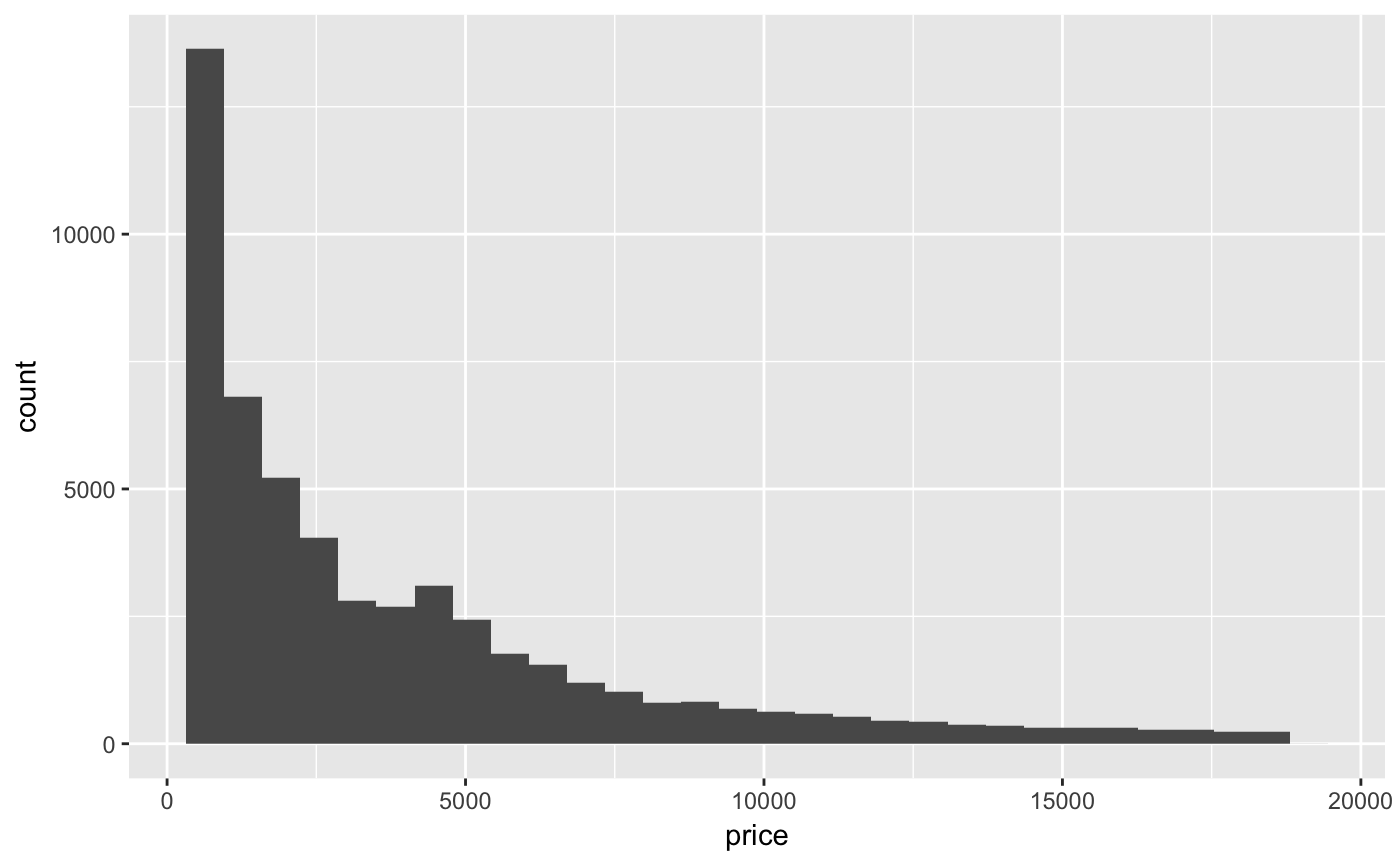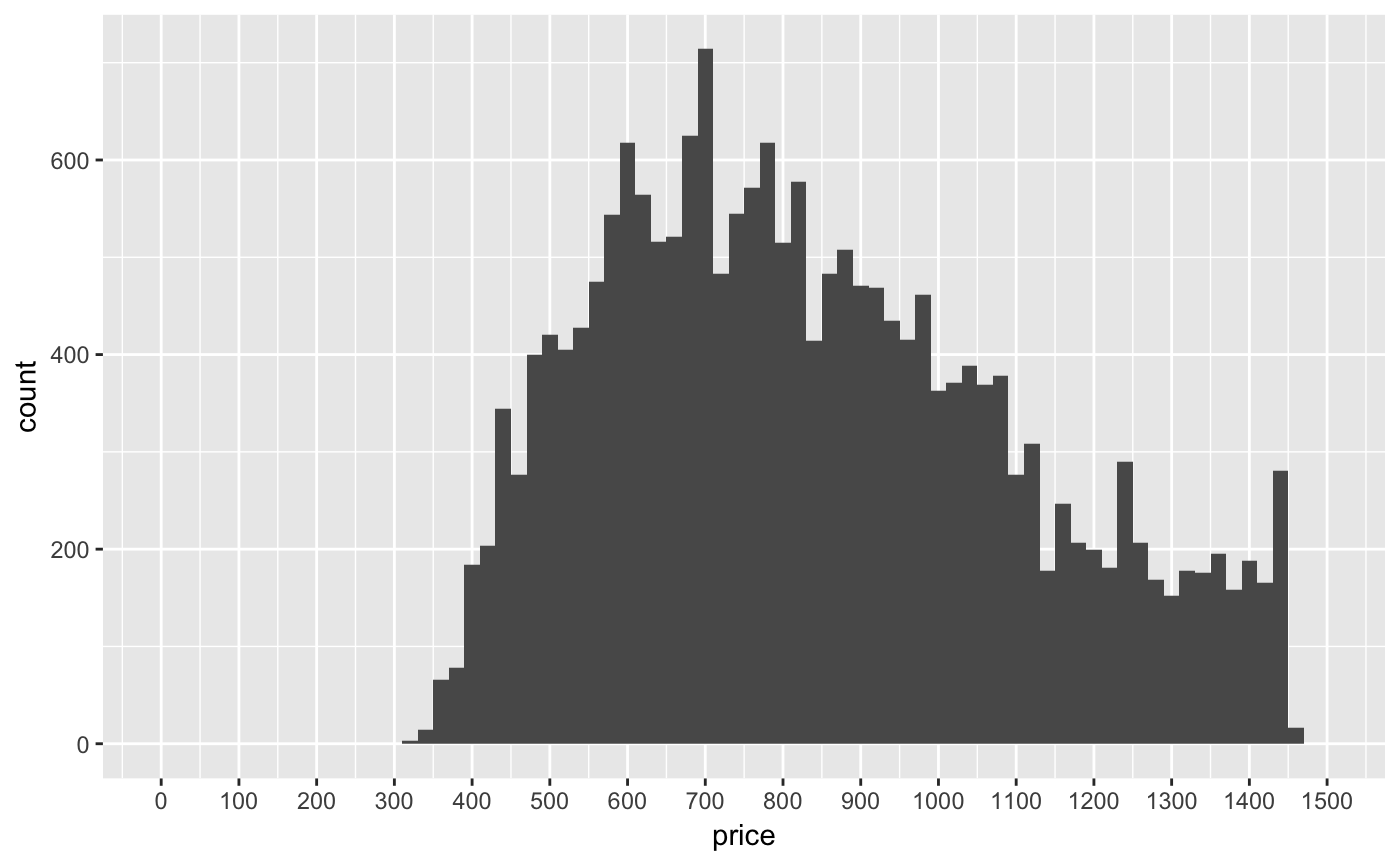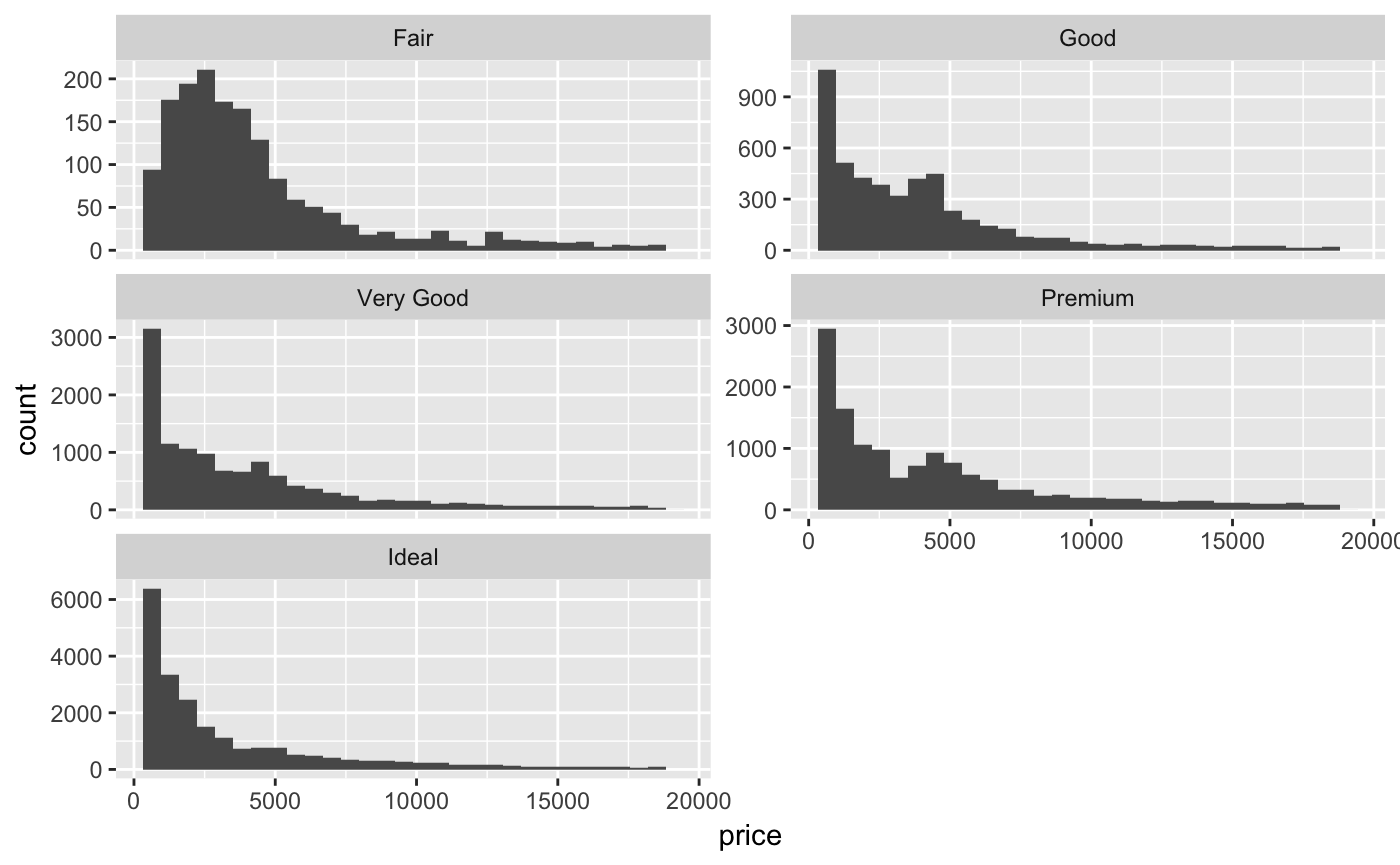The ‘diamonds’ dataset is one of the datasets provided with the ggplot2 R package. We’re going to see if we can predict the price of a diamond based on its characteristics.

We will first conduct an EDA to get to know the data and analyze the impact of the different variables. We will then push the analysis further in order to build a linear model and use it to predict prices.

NOTE: This notebook contains two analyses condensed in one. While they definitely are related, there are a few points that need to be revised to help the reader follow the logic easily. This will be done in the near future, but we found that this notebook was interesting enough to be published in its raw form, waiting for revision.

``````library(ggplot2)
library(GGally)
library(scales)
library(memisc)``````
``````Loading required package: lattice

Attaching package: ‘memisc’

The following object is masked from ‘package:scales’:

percent

The following objects are masked from ‘package:stats’:

contr.sum, contr.treatment, contrasts

The following object is masked from ‘package:base’:

as.array``````
``````library(RColorBrewer)
data("diamonds")``````

# Univariate Analysis

## General Information

Dimensions of the dataset:

``dim(diamonds)``
`` 53940    10``

Name of the variables:

``str(diamonds)``
``````Classes ‘tbl_df’, ‘tbl’ and 'data.frame':   53940 obs. of  10 variables:
\$ carat  : num  0.23 0.21 0.23 0.29 0.31 0.24 0.24 0.26 0.22 0.23 ...
\$ cut    : Ord.factor w/ 5 levels "Fair"<"Good"<..: 5 4 2 4 2 3 3 3 1 3 ...
\$ color  : Ord.factor w/ 7 levels "D"<"E"<"F"<"G"<..: 2 2 2 6 7 7 6 5 2 5 ...
\$ clarity: Ord.factor w/ 8 levels "I1"<"SI2"<"SI1"<..: 2 3 5 4 2 6 7 3 4 5 ...
\$ depth  : num  61.5 59.8 56.9 62.4 63.3 62.8 62.3 61.9 65.1 59.4 ...
\$ table  : num  55 61 65 58 58 57 57 55 61 61 ...
\$ price  : int  326 326 327 334 335 336 336 337 337 338 ...
\$ x      : num  3.95 3.89 4.05 4.2 4.34 3.94 3.95 4.07 3.87 4 ...
\$ y      : num  3.98 3.84 4.07 4.23 4.35 3.96 3.98 4.11 3.78 4.05 ...
\$ z      : num  2.43 2.31 2.31 2.63 2.75 2.48 2.47 2.53 2.49 2.39 ...``````

Summary:

``summary(diamonds)``
``````     carat               cut        color        clarity          depth           table           price
Min.   :0.2000   Fair     : 1610   D: 6775   SI1    :13065   Min.   :43.00   Min.   :43.00   Min.   :  326
1st Qu.:0.4000   Good     : 4906   E: 9797   VS2    :12258   1st Qu.:61.00   1st Qu.:56.00   1st Qu.:  950
Median :0.7000   Very Good:12082   F: 9542   SI2    : 9194   Median :61.80   Median :57.00   Median : 2401
Mean   :0.7979   Premium  :13791   G:11292   VS1    : 8171   Mean   :61.75   Mean   :57.46   Mean   : 3933
3rd Qu.:1.0400   Ideal    :21551   H: 8304   VVS2   : 5066   3rd Qu.:62.50   3rd Qu.:59.00   3rd Qu.: 5324
Max.   :5.0100                     I: 5422   VVS1   : 3655   Max.   :79.00   Max.   :95.00   Max.   :18823
J: 2808   (Other): 2531
x                y                z
Min.   : 0.000   Min.   : 0.000   Min.   : 0.000
1st Qu.: 4.710   1st Qu.: 4.720   1st Qu.: 2.910
Median : 5.700   Median : 5.710   Median : 3.530
Mean   : 5.731   Mean   : 5.735   Mean   : 3.539
3rd Qu.: 6.540   3rd Qu.: 6.540   3rd Qu.: 4.040
Max.   :10.740   Max.   :58.900   Max.   :31.800
``````

Levels of our cateogricalvariables:

``levels(diamonds\$cut)``
`` "Fair"      "Good"      "Very Good" "Premium"   "Ideal"    ``
``levels(diamonds\$color)``
`` "D" "E" "F" "G" "H" "I" "J"``
``levels(diamonds\$clarity)``
`` "I1"   "SI2"  "SI1"  "VS2"  "VS1"  "VVS2" "VVS1" "IF"  ``

# Univariate analysis

Let’s jump right into it and focus our analysis on price.

## Price histogram

``qplot(data = diamonds, x = price)````summary(diamonds\$price)``
``````   Min. 1st Qu.  Median    Mean 3rd Qu.    Max.
326     950    2401    3933    5324   18823 ``````

The distribution of diamonds prices is clearly right-skewed.

## Diamond price detail

Let’s get some numbers:

``sum(diamonds\$price < 500)``
`` 1729``
``sum(diamonds\$price < 250)``
`` 0``
``sum(diamonds\$price >= 15000)``
`` 1656``

Our dataset contains: - 1729 diamonds with a price below \$500 - 0 diamonds with a price below \$250 - 15,000 diamonds with a price equal to or above \$15,000

## Histogram - cheaper diamonds

Let’s get a look at the cheapest diamonds:

``````qplot(data = diamonds, x = price,
binwidth = 20) +
scale_x_continuous(limits = c(0, 1500), breaks = seq(0, 1500, 100))````````````Mode <- function(x) {
ux <- unique(x)
ux[which.max(tabulate(match(x, ux)))]
}
Mode(diamonds\$price)``````
`` 605``

The mode of the cheapest diamonds (with a price between \$0 and \$1,500) is 605.

# Bivariate analysis

## Faceting - Histogram of diamond prices by cut

Let’s facet our prices by the quality of the cut.

## Scaling - Histogram of diamond prices by cut

``````qplot(data = diamonds, x = price) +
facet_wrap(~cut, ncol= 2, scales = 'free_y')````````by(diamonds\$price, diamonds\$cut, summary)``
``````diamonds\$cut: Fair
Min. 1st Qu.  Median    Mean 3rd Qu.    Max.
337    2050    3282    4359    5206   18574
---------------------------------------------------------------------------------------------
diamonds\$cut: Good
Min. 1st Qu.  Median    Mean 3rd Qu.    Max.
327    1145    3050    3929    5028   18788
---------------------------------------------------------------------------------------------
diamonds\$cut: Very Good
Min. 1st Qu.  Median    Mean 3rd Qu.    Max.
336     912    2648    3982    5373   18818
---------------------------------------------------------------------------------------------
``````qplot(data = diamonds, x = price / carat, binwidth = 0.1) +